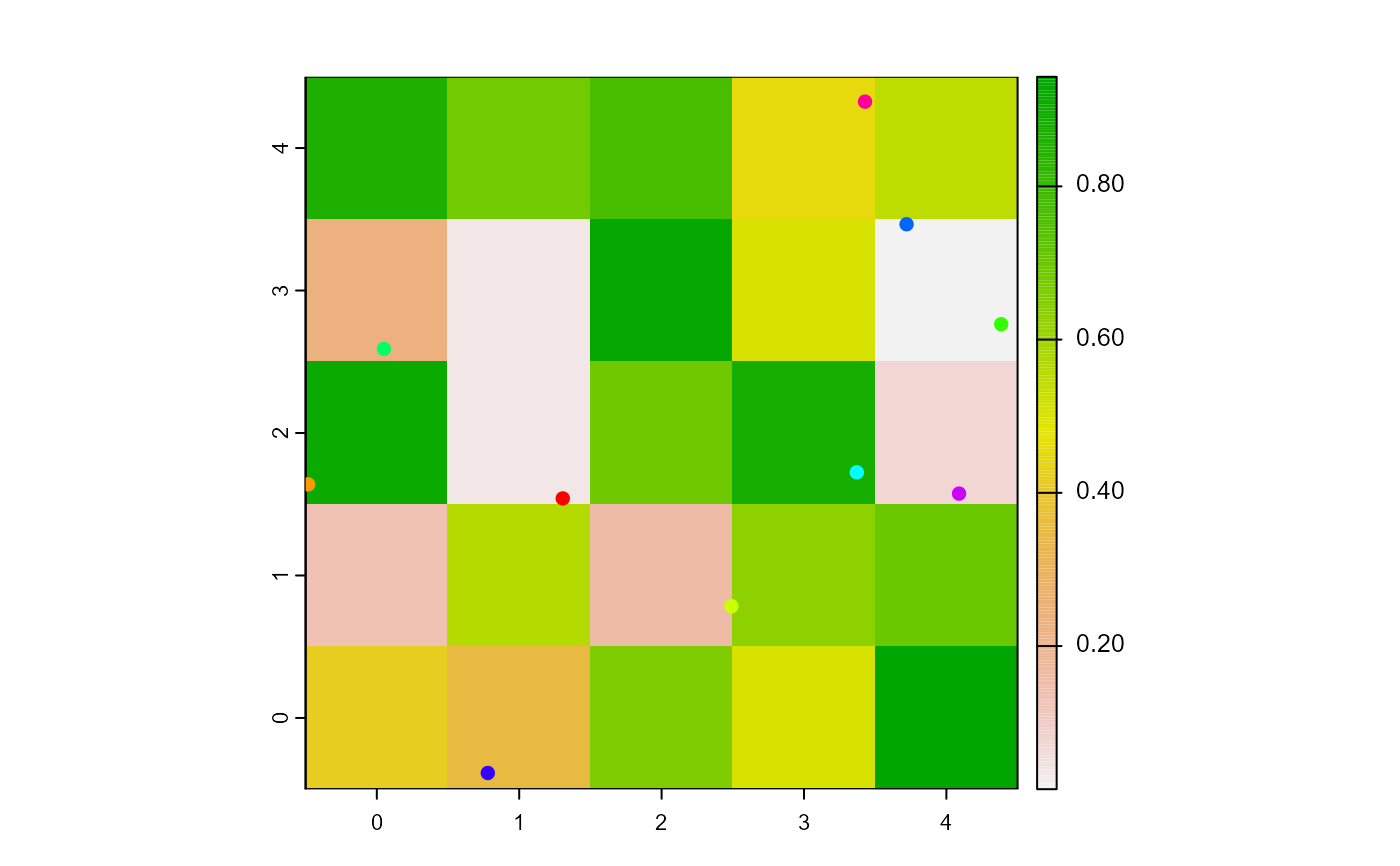Report n random xcor coordinates within the world's extent.

randomXcor(world, n)

# S4 method for worldNLR,numeric
randomXcor(world, n)

## Arguments

world

WorldMatrix or worldArray object.

n

Integer.

## Value

Numeric. Vector of length n of xcor coordinates.

Sarah Bauduin

## Examples

w1 <- createWorld(minPxcor = 0, maxPxcor = 4, minPycor = 0, maxPycor = 4,
data = runif(25))
t1 <- createTurtles(n = 10, coords = cbind(xcor = randomXcor(world = w1, n = 10),
ycor = randomYcor(world = w1, n = 10)))
plot(w1)
points(t1, col = of(agents = t1, var = "color"), pch = 16)